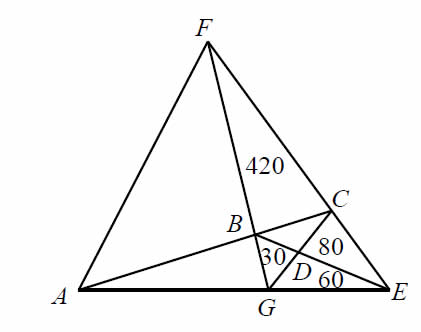# find the area

Geometry Level 4A triangle is divided into seven triangles. The areas of four of them are $420 cm^2, 80 cm^2, 60 cm^2$ and $30 cm^2$ as shown in the diagram on the right. Find the area of $\triangle AEF$, in $cm^2$

×

Problem Loading...

Note Loading...

Set Loading...